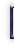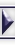## Special Method Attributes in Python

#### By Pramode C E

C++ programmers use 'operator overloading' to apply built-in operators to user defined classes. Thus, a complex number class may have an addition operator which makes it possible for us to use two objects of type 'complex' in an arithmetic expression in the same way we use integers or floating point numbers. The Python programming language provides much of the same functionality in a simple and elegant manner - using special method attributes. This is a quick introduction to some of the special methods through code snippets which we wrote while trying to digest the Python language reference. The code has been tested on Python ver 1.5.1.

## Classes and objects in Python

Let us look at a simple class definition in Python:

```
class foo:
def __init__(self, n):
print 'Constructor called'
self.n = n

def hello(self):
print 'Hello world'

def change(self, n):
print 'Changing self.n'
self.n = n

f = foo(10)  # create an instance of class foo with a data field 'n' whose value is 10.
print f.n    # prints 10. Python does not support member access control the way C++ does.
f.m = 20     # you can even add new fields!
f.hello()    # prints 'Hello world'
foo.change(f, 40)
print f.n    # prints 40

```
The method __init__ is similar to a 'constructor' in C++. It is a 'special method' which is automatically called when an object is being created.

We see that all member functions have a parameter called 'self'. Now, when we call f.hello(), we are actually calling a method belonging to class foo with the object 'f' as the first parameter. This parameter is usually called 'self'. Note that it can be given any other name, though you are encouraged to name it that way by convention. It is even possible to call f.hello() as foo.hello(f). C++ programmers will find some relation between 'self' and the keyword 'this'(in C++) through which the hidden first parameter to member function invocations can be accessed.

Consider the following class definition:
```
class foo:
def __init__(self, n):
self.n = n
t = foo(0)
t.n = self.n + right.n
return t

```
Now we create two objects f1 and f2 of type 'foo' and add them up:
```
f1 = foo(10)  # f1.n is 10
f2 = foo(20)  # f2.n is 20
f3 = f1 + f2
print f3.n    # prints 30

```
What happens when Python executes f1+f2 ? In fact, the interpreter simply calls f1.__add__(f2). So the 'self' in function __add__ refers to f1 and 'right' refers to f2.

Let us look at another flavor of the __add__ special method:
```
class foo:
def __init__(self, n):
self.n = n
t = foo(0)
t.n = self.n + left.n
print 'left.n is', left.n
return t

f1 = foo(10)
f2 = foo(20)
f3 = f1 + f2  # prints 'left.n is 10'

```
The difference in this case is that f1+f2 is converted into f2.__radd__(f1).

## How objects print themselves - the __str__ special method

```
class foo:
def __init__(self, n1, n2):
self.n1 = n1
self.n2 = n2

def __str__(self):
return 'foo instance:'+'n1='+`self.n1`+','+'n2='+`self.n2`

```
class foo defines a special method called __str__. We will see it in action if we run the following test code:
```
f1 = foo(10,20)
print f1    # prints 'foo instance: n1=10,n2=20'

```
The reader is encouraged to look up the Python Language Reference and see how a similar function, __repr__ works.

## Truth value testing with __nonzero__

__nonzero__ is called to implement truth value testing. It should return 0 or 1. When this method is not defined, __len__ is called, if it(__len__) is defined. If a class defines neither __len__ nor __nonzero__, all its instances are considered true. This is how the language reference defines __nonzero__.

Let us put this to test.

```
class foo:
def __nonzero__(self):
return 0

class baz:
def __len__(self):
return 0

class abc:
pass

f1 = foo()
f2 = baz()
f3 = abc()

if (not f1): print 'foo: false'  # prints 'foo: false'
if (not f2): print 'baz: false'  # prints 'baz: false'
if (not f3): print 'abc: false'  # does not print anything

```

## The magic of __getitem__

How would you like your object to behave like a list? The distinguishing feature of a list (or tuple, or what is in general called a 'sequence' type) is that it supports indexing. That is, you are able to write things like 'print a[i]'. There is a special method called __getitem__ which has been designed to support indexing on user defined objects. Here is an example:
```
class foo:
def __init__(self, limit):
self.limit = limit

def __getitem__(self, key):
if ((key > self.limit-1) or (key < -(self.limit-1))):
raise IndexError
else:
return 2*key

f = foo(10)       # f acts like a 20 element array
print f, f  # prints 0, 2
print f[-3]       # prints -6
print f       # generates IndexError

```
There are some additional methods available like __setitem__, __delitem__, __getslice__, __setslice__, __delslice__ .

## Attribute access with __getattr__

__getattr__(self,name) is called when attribute lookup fails to find the attribute whose name is given as the second argument. It should either raise an AttributeError exception or return a computed attribute value.
```
class foo:
def __getattr__(self, name):
return 'no such attribute'

f = foo()
f.n = 100
print f.n     # prints 100
print f.m     # prints 'no such attribute'

```
Note that we also have a builtin function getattr(object, name). Thus, getattr(f, 'n') returns 100 and getattr(f,'m') returns the string 'no such attribute'. It is easy to implement stuff like delegation using getattr. Here is an example:
```
class Boss:
def __init__(self, delegate):
self.d = delegate

def credits(self):
print 'I am the great boss, I did all that amazing stuff'

def __getattr__(self, name):
return getattr(self.d, name)

class Worker:
def work(self):
print 'Sigh, I am the worker, and I get to do all the work'

w = Worker()
b = Boss(w)
b.credits()  # prints 'I am the great boss, I did all that amazing stuff'
b.work()     # prints 'Sigh, I am the worker, and I get to do all the work'

```